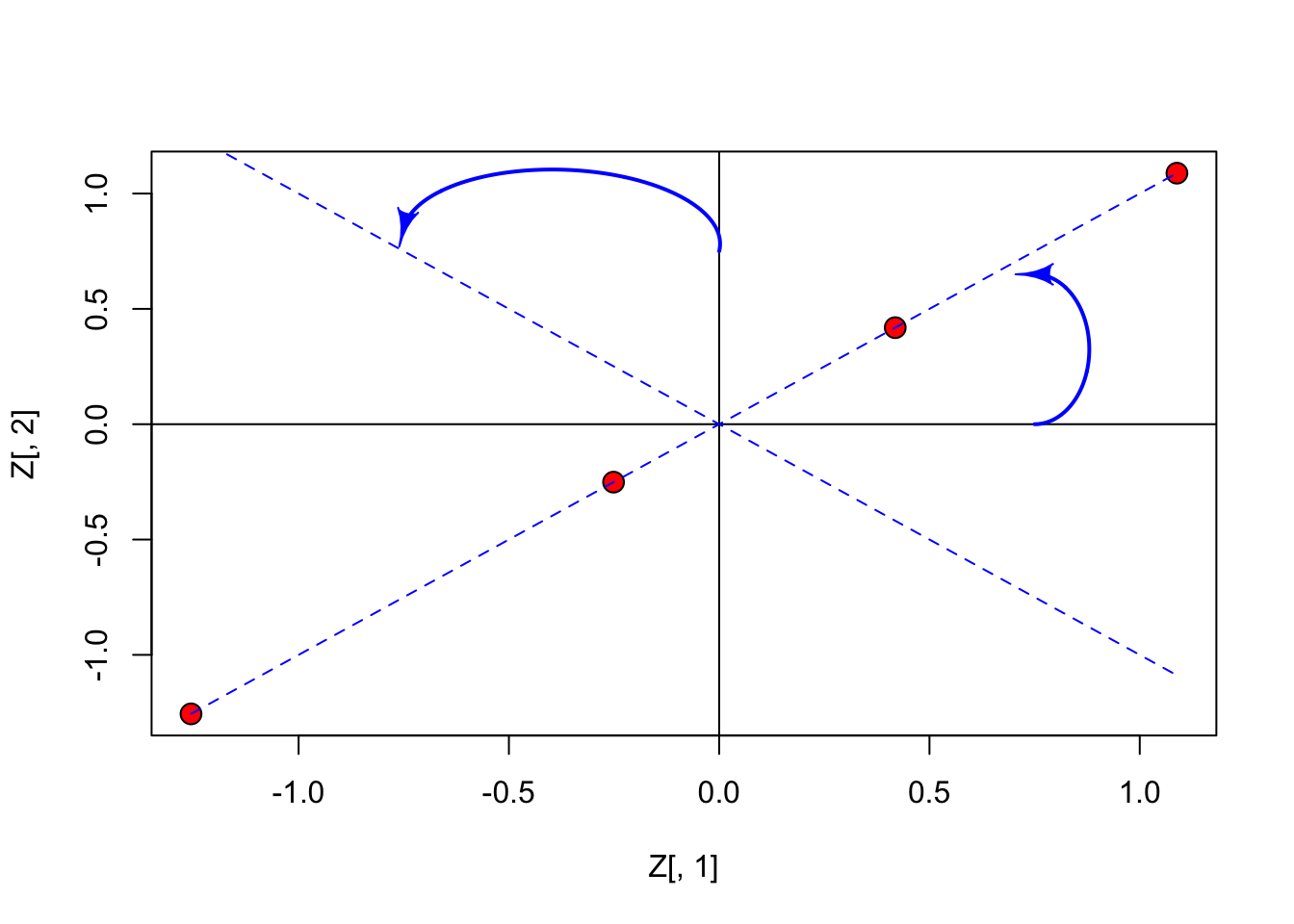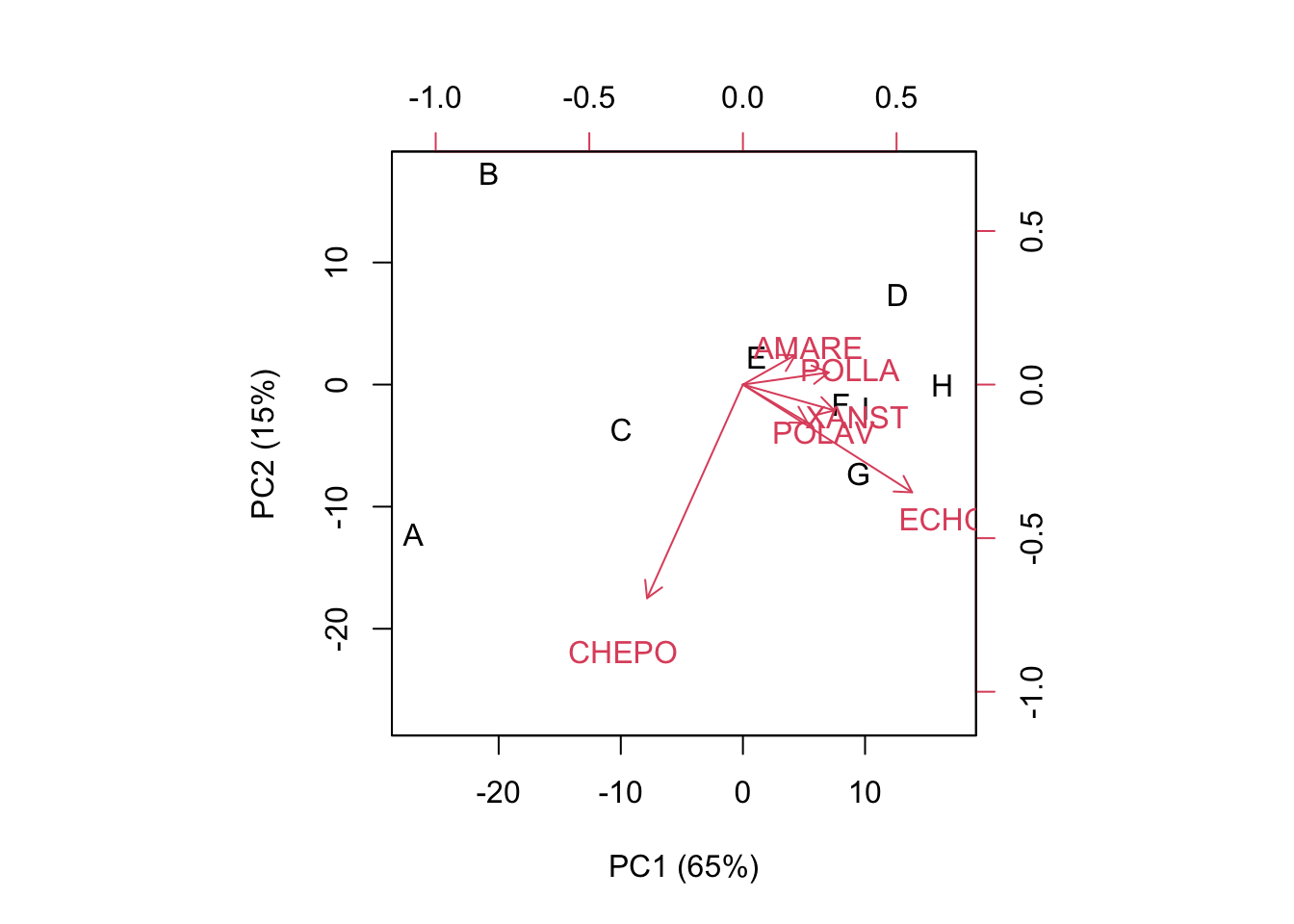# Principal Component Analysis: a brief intro for biologists

Andrea Onofri ·  Added on November 23, 2021 ·  24 min read

In this post I am revisiting the concept of Principal Component Analysis (PCA). You might say that there is no need for that, as the Internet is full with posts relating to such a rather old technique. However, I feel that, in those posts, the theoretical aspects are either too deeply rooted in maths or they are skipped altogether, so that the main emphasis is on interpreting the output of an R function. I think that both approaches may not be suitable for biologists: the first one may be too difficult to understand, while skipping altogether the theoretical aspects promotes the use of R as a black-box, which is dangerouse for teaching purposes. That’s why I wrote this post… I wanted to make my attempt to create a useful lesson. You will tell me whether I suceeded or not.

# What is Principal Component Analysis?

A main part of field experiments is multivariate, in the sense that several traits are measured in each experimental unit. For example, think about yield quality in genotype experiments or about the composition of weed flora in herbicide trials: in both cases, it is very important to consider several variables altogether, otherwise, we lose all information about the relationships among the observed variables and we lose the ability of producing a convincing summary of results.

Multivariate methods can help us to deal with multivariate datasets. One main task of multivariate analysis is ordination, i.e. organising the observations so that similar subjects are near to each other, while dissimilar subjects are further away. Clearly, ordination is connected to ‘representation’ and it is aided by techniques that permit a reduction in the number of variables, with little loss in terms of descriptive ability.

Principal Component Analysis (PCA) is one of these techniques. How can we reduce the number of variables? How can we use, say, two variables instead of six, without losing relevant information? One possibility is to exploit the correlations between pairs of variables: whenever this is high, both variables carry a similar amount of information and there may be no need of using both of them.

Let’s make a trivial example: think about four subjects where we measured two variables (X1 and X2), with the second one being exactly twice as much the first one. As we know, these four subjects and their characteristics take the form of a 4 x 2 matrix, which is shown below.

rm(list=ls())
X <- c(12, 15, 17, 19)
Y <- c(24, 30, 34, 38)
dataMat <- data.frame(X, Y, row.names=letters[1:4])
print(dataMat)
##    X  Y
## a 12 24
## b 15 30
## c 17 34
## d 19 38

As I said, this example is trivial: it is indeed totally clear that the second variable does not add any additional information with respect to the first one, and thus we could as well drop it without hindering the ordination of the four subjects. Nevertheless, naively dropping a variable may not be optimal whenever the correlation is less then perfect! What should we do, then?

First of all, we should note that the two variables have different means and standard deviations. Thus, we might like to center and, possibly, standardise them. The first practice is necessary, while the second one is optional and it may dramatically change the interpretation of results. Therefore, when you write your paper, please always inform the readers whether you have standardised or not! When we work on centered (not standardised) data, we usually talk about PCA on covariance matrix, while, when we work on standardised data, we talk abot PCA on correlation matrix. The reason for these namings will be clear later on.

Let’s standardise the data, and represent them in the Euclidean space: in this specific case the points (subjects) lie along the bisector of first and third quadrant (all points have equal co-ordinates).

Z <- scale(dataMat, scale=T, center=T)[1:4,1:2]
Z
##            X          Y
## a -1.2558275 -1.2558275
## b -0.2511655 -0.2511655
## c  0.4186092  0.4186092
## d  1.0883839  1.0883839## PCA: a ‘rotation’ procedure

From the previous graph we may note that it would be useful to rotate the axes by an angle of 45°; in this case, the points will lie along the x-axis and they would all have a null co-ordinate on the y-axis. As the consequence, this second dimension would be totally useless.

Rotating the axes is a matrix multiplication problem: we have to take the $$Z$$ and multiply it by a rotation matrix, to get the co-ordinates of subjects in the new reference system. We do not need the details: if we want to rotate by an angle $$\alpha$$, the rotation matrix is:

$V = \left[\begin{array}{cc} \cos \alpha & - \sin \alpha \\ \sin \alpha & \cos \alpha \end{array} \right]$

In our case ($$\alpha = 45° = \pi/4$$), it is:

$V = \left[\begin{array}{cc} \frac{\sqrt{2}}{2} & - \frac{\sqrt{2}}{2} \\ \frac{\sqrt{2}}{2} & \frac{\sqrt{2}}{2} \end{array} \right]$

## PCA: a eigenvalue decomposition procedure

How do we get the rotation matrix, in general? We take the correlation matrix and submit it to eigenvalue decomposition:

corX <- cor(dataMat)
eig <- eigen(corX)
eig
## eigen() decomposition
## $values ##  2 0 ## ##$vectors
##           [,1]       [,2]
## [1,] 0.7071068 -0.7071068
## [2,] 0.7071068  0.7071068
V <- pca$vectors row.names(V) <- colnames(WeedPop[,2:7]) colnames(V) <- paste("PC", 1:6, sep="") U <- C %*% V print(U, digits = 3) ## PC1 PC2 PC3 PC4 PC5 PC6 ## A -26.97 -12.2860 3.129 -0.271 -0.00398 -0.581 ## B -20.81 17.3167 -2.935 3.081 -1.29287 -0.475 ## C -9.95 -3.6671 3.035 -0.819 2.38378 0.801 ## D 12.69 7.3364 11.756 -3.732 -1.45392 0.180 ## E 1.13 2.2854 -5.436 -3.412 1.86926 2.247 ## F 8.05 -1.6470 -0.455 -2.895 0.22101 -1.607 ## G 9.46 -7.3174 -1.213 5.072 -4.98975 0.976 ## H 16.34 -0.0632 0.799 7.213 4.07606 -0.509 ## I 10.07 -1.9578 -8.680 -4.237 -0.80960 -1.032 print(V, digits = 3) ## PC1 PC2 PC3 PC4 PC5 PC6 ## POLLA 0.349 0.0493 0.5614 0.1677 0.5574 -0.4710 ## CHEPO -0.390 -0.8693 0.2981 0.0013 0.0480 0.0327 ## ECHCG 0.688 -0.4384 -0.3600 -0.4322 -0.0113 -0.1324 ## AMARE 0.213 0.1200 0.6808 -0.4155 -0.4893 0.2547 ## XANST 0.372 -0.1050 0.0499 0.4107 0.2631 0.7813 ## POLAV 0.263 -0.1555 0.0184 0.6662 -0.6150 -0.2903 print(d, digits = 3) ##  243.01 72.93 34.13 17.49 6.90 1.39 One important difference is that the variance of components in the subject-score matrix has a sum equal to the sum of variances on the original matrix X: sum( apply(X, 2, var) ) ##  375.8411 Another important difference is that the inner products return the values in the centered matrix, i.e. the weed coverings of the different species as differences with respect to column-means. The goodness of representation and biplot are very similar to the previous ones. However, we may note that the weeds with higher variances (CHEPO and ECHCG) tend to have higher weight on the ordination, as shown by longer vectors. cumsum(d)/sum(d) * 100 ##  64.65801 84.06118 93.14164 97.79412 99.62927 100.00000 apply(X, 2, var) ## POLLA CHEPO ECHCG AMARE XANST POLAV ## 43.45750 95.11111 136.86111 32.61111 38.73528 29.06500 biplot(U[,1:2], V[,1:2], xlab="PC1 (65%)", ylab="PC2 (15%)")# PCA with R PCA can be easily performed with R, altough there are several functions, among which it is often difficult to make a selection. We will now briefly introduce only prcomp() and princomp(), both in the ‘stats’ package. These two functions are different in relation to the algorithm that is used for calculation (spectral decomposition and singular value decomposition). In princomp(), we need to use the argument ‘scale’ to select between a PCA on centered data (‘scale = F’) and a PCA on standardised data (‘scale = T’). In prcomp(), we can make the same selection by using the argument ‘cor’. Other differences relate to the fact that prcomp() shows the standard deviations of subject-scores, instead of their variances. Please, note that the output of princomp() is slightly different, as this function operates on standardised data by using population-based standard deviations and not sample-based standard deviations. This might be preferable, as PCA is, fundamentally, a descriptive method. #prcomp() pcaAnalysis<-prcomp(X, scale=T) summary(pcaAnalysis) print( pcaAnalysis$x, digits=3) #Subject-scores
print(pcaAnalysis$rotation, digits=3) #Trait-scores #princomp() pcaAnalysis2 <- princomp(X, cor=T) print(pcaAnalysis2, digits=3) print(pcaAnalysis2$scores, digits=3)
print(pcaAnalysis2\$loadings, digits=3)

Prof. Andrea Onofri
Department of Agricultural, Food and Environmental Sciences
University of Perugia (Italy)

2022 (6)
2021 (16)
2020 (14)
2019 (14)
2018 (2)
2014 (1)